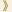HomeShaw Laureates2014Mathematical SciencesEssay

For more than two hundred years, symmetry groups have been at the centre of mathematics and its applications: in Fourier’s work on the heat equation in the early 1800s; in the work of Weyl and Wigner on quantum mechanics in the early 1900s; and in the approach to number theory created by Artin and Chevalley. These classical works show that answers to almost any question involving a symmetry group lie in understanding its realizations as a group of linear transformations, that is, in terms of its representations.

Lusztig’s work has completely transformed our understanding of representation theory, providing complete and precise answers to fundamental questions that were understood before only in very special cases.  What he has done has advanced all of the mathematics where symmetry groups play a role: from Langlands’ programme for understanding automorphic forms in number theory, to classical problems of harmonic analysis on real Lie groups.

Here are some hints of the ideas at the heart of Lusztig’s work. The most basic symmetry group in mathematics is GL(n), the group of invertible n ×n matrices. (The entries in the matrices will be different in different problems.) One of the most important invariants of a matrix is the collection of its n eigenvalues. The eigenvalues do not come with an ordering, so they can be rearranged using the permutation group Sn (consisting of the n! permutations of (1, 2, …, n)). A consequence (understood already in the nineteenth century) is that some of the properties of the (large and complicated) group GL(n) can be encoded by the (smaller and simpler) group Sn.

All permutations of (1, …, n) may be built up from n-1 “simple transpositions”: the permutations sithat exchange i with i+1, and leave everything else alone.  For example, we can reverse the order of (1, 2, 3) by first exchanging the 1 and the 2, then exchanging the 2 and the 3, and finally exchanging the 1 and the 2 again:

(1,2,3) →(2,1,3) →(3,1,2) →(3,2,1).

Elementary results from linear algebra provide a beautiful decomposition of GL(n) into pieces labelled by permutations; these are essentially the Schubert varieties for GL(n).  The piece labelled by the trivial permutation consists of the upper triangular matrices, where all problems of linear algebra are easy. The piece labeled by the simple transposition siconsists of matrices with a single nonzero entry below the diagonal, in column i and row (i+1). As the permutation gets larger, the corresponding matrices become less and less upper triangular, and the corresponding Schubert variety gets more and more complicated.

A first theme in Lusztig’s work is that the algebraic subtleties of representation theory correspond perfectly to the topological and geometric subtleties of Schubert varieties.  Although this idea is foreshadowed in earlier works, such as that of Borel and Weil in the 1950s on representations of compact groups, Lusztig’s results have revolutionized the field.  Mathematicians talk about geometric representation theory to distinguish what is possible now from its antecedents.

Representations are complicated, as are the Schubert varieties to which Lusztig’s work relates them. A second theme in his work is the creation of combinatorial tools — easy to describe, but almost without precedent in mathematics — to describe in fantastic detail the topology and geometry of Schubert varieties. This theme begins in a 1979 paper with David Kazhdan, and continues through Lusztig’s most recent work.  The idea is to build complicated Schubert varieties from simpler ones exactly as complicated permutations are built from simpler ones, by multiplication of simple transpositions.  This description of permutations has been studied in combinatorics for more than a hundred years, but Lusztig’s ideas have opened whole new fields of investigation for them.

A third theme in Lusztig’s work is the new field of quantum groups, introduced by Vladimir Drinfeld in the 1980s. Lusztig has said that the test of a new theory is whether you can use it to answer questions that were asked before the new theory existed.  He has made quantum groups pass that test in a number of amazing ways. For example, his theory of canonical bases (which can be introduced only using quantum groups) allowed him to extend the classical theory of “totally positive matrices” from GL(n) to all reductive groups.

There is much more to say about Lusztig’s work: on modular representation theory, on affine Hecke algebras, and on p-adic groups, for instance. He has touched widely separated parts of mathematics, reshaping them and knitting them together.  He has built new bridges from representation theory to combinatorics and algebraic geometry, solving classical problems in those disciplines and creating exciting new ones.  This is a remarkable career, as exciting to watch today as it was at the beginning more than forty years ago.

Mathematical Sciences Selection Committee
The Shaw Prize

24 September 2014   Hong Kong